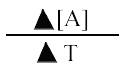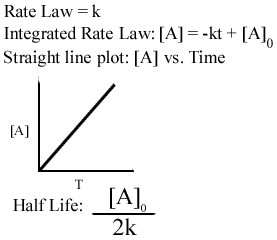MainTutorialsOrganic ChemistryPractice TestsOnline QuizzesReference ToolsKinetics
By Hao Zhang

I tried to make this tutorial as straightforward as possible. The bolded words are terms that you need to know. While standard test do not require you to memorize the equations, knowing them will only help you.

• Chemical kinetics- the area of chemistry that studies the rates of reactions
• Reaction mechanism- the steps involved in a chemical reaction.

With the understanding of the speed and the process of a reaction, chemists can facilitate reactions to their needs.

Reaction rate is simply the change in the amount of product or reactant over time. The amount is measured in molarity while the change in time is in seconds. Therefore the reaction rate is:The triangle in front of the letters is called "delta" and represents "change of."

A is the reactant or product, and the enclosed brackets stands for the molarity. Think of the reaction rate as the slope of the concentration vs. time graph.

• The reaction rate of the reactant is always negative because the reactant is decreasing to form the product.
• The reaction rate of the product is always positive because the product is being formed.

Ex. N2(g)+ 3H2(g) Ţ 2NH3(g)

The reaction rate is: -D [N2 ]/D T. The rate of consumption of hydrogen is 1.5 times as fast as the formation of ammonia because 3 moles of hydrogen will produce 2 moles of ammonia.

A reaction can proceed forward as well as backwards. For now the reverse reaction is considered negligible because the reactions in this tutorial are studied under conditions where the reverse reaction is insignificant.The rate law, another form of is: Rate = k[A]n

Where k is the rate constant and n is the order, both values must be determined through experiments.

For the rate law to hold true:

• We assume that the reverse reaction is insignificant.
• The order, n, is an experimental value.

The rate law of N2(g)+ 3H2(g) Ţ 2NH3(g)

Rate = k[N2]n[3H2]m

The overall reaction order is the total order in the equation: m + n

Again, n and m are experimental figures.

There are two types of rate laws.

Differential rate law- relates the rate law with the concentration of reactant. Ex. Rate = k[A]n

Integrated rate law- relates the rate law with time. For those of you who have studied calculus, this law is the integration of the differential rate law. Ex. ln[A]=-kt+[A]0

Three types of rate orders.

0 order
the rate of the reaction is independent of the concentration. The rate is constant.Half-life is the amount of time it takes for something to decompose to half of its original amount. With a half-life of 5 days, 1kg of road kill would become 0.5 kg in 5 days.First order
the concentration of reaction is directly proportional to the rate. If you triple the concentration, the reaction rate will triple.Second order
the reaction rate grows exponentially with concentration. If you double the concentration, the reaction rate quadruples.

Since a reaction occurs in a series of steps, names are given to each step along with the substances involved in the reaction.

O3(g) Ţ O2(g) + O(g)

O3(g) + O(g) Ţ 2 O2(g)

O(g) in called an intermediate.

• An intermediate does not qualify as a reactant or product, but its consumed or formed.
• Each step of reaction is called an elementary step.
• Molecularity- minimum number of molecules that must collide for the reaction in that step to take place. Here is how you name them:
 Number of reactants Name Rate Law 1;[A]Ţ products Unimolecular k[A] 2;2[A]Ţ products Bimolecular k[A]2 2;[A]+[B]Ţ products Bimolecular k[A][B] 3;2[A]+[B]Ţ products Termolecular k[A] 2 [B] 3;[A]+[B]+[C]Ţ products Termolecular k[A][B][C]

So what does temperature have on the reaction rate? For a reaction to occur, molecules must collide, and raising the temperature increases the movement of molecules. Therefore, as temperature increases, the reaction rate also increases.

But the for a reaction to proceed, a specific amount of energy must first be achieved. This energy is called activation energy. A rise in temperature also lowers the activation energy.

Pg. 572 fig. 12.9

Pg. 573 fig. 12.10

For a reaction to occur:

• Molecules must produce enough energy to overcome the activation energy.
• The molecules in the reactants must be orientated in such a manner that old bonds are broken so new bonds in the product can form.The most common equation for activation energy is:

Learn this equation, it’s very useful. After all, anybody can plug in numbers and use a calculator.

R=8.3145

A catalyst is a substance that speeds up chemical reaction without being consumed in the process. A prime example is the enzymes in the human body. Chemists use catalysts to speed up slow reactions. An example of this is the production of ammonia, know as the Haber Process.

A catalyst helps a reaction by lowering its activation energy; therefor, speeding up the reaction. Since the reaction is very slow on its own, chemists add a catalyst to speed it up.

Two types of catalyst:

Homogeneous-the catalyst exists in the same phase during the reaction.

O3(g) Ţ O2(g) + O(g)

O3(g) + O(g) Ţ 2 O2(g)

For this reaction, the O(g) is the catalyst.

Heterogeneous-the catalyst enters and exists in different phases of the reaction. The catalyst usually provided the area where gaseous molecules can adsorb on its surface, then it forms the product.

The term adsorption is usually related with heterogeneous catalysts. Adsorption is the collection of a substance on the surface of another.news | about us | contact us tutorials index | organic chemistry | practice tests | online quizzes | reference tools site copyright (c) 2002-2013 Learn ChemSubscribe to our low volume newsletter to receive up-to-date information about the CHEM SITE